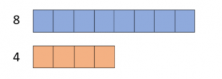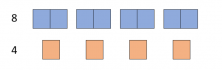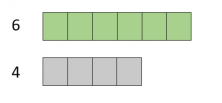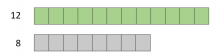Smartick is an online platform for children to master math in only 15 minutes a day

Feb02

# Let’s Learn about Ratios with Singaporean Bar Models

In today’s post, we’re going to study the concept of ratios using the “Singapore Bar Model” methodology.

First, we need to take into consideration that two quantities can be compared in different ways. By subtracting, by a fraction, and by multiplying…but today, we’re going to use ratios as a factor of comparison.

To be able to compare two or more quantities by using ratios, they need to be expressed in the same unit. Once we have the quantities expressed in the same unit, we need to find one of the quantities with respect to the other.

### Let’s look at an example:

“John and Paul are twin brothers. Their mom bought them a bag of muffins that has 4 identical muffins. What is the muffin ratio for the brothers?”The muffin ratio in respect to the number of boys is 2 muffins for each boy and it’s written as (2:1). The relationship is bidirectional, it can also be (1:2), which means that there is one boy for every 2 muffins.

Now, we’re going to apply this to the Singapore Bar Models:

What is the ratio of 4 orange units for 8 blue units?The answer is the same as the one from the previous example.1:2 (there is 1 orange unit for every 2 blue units) and 2:1 (there are 2 blue units for every orange unit)Now, let’s look at a different case where the two quantities aren’t divisible by each other:Here, we see a direct relationship; the ratio between these two quantities isn’t divisible by one another. 6:4 (6 green units for every 4 grey units) and 4:6 (4 grey units for every 6 green units).

Now, we’re going to check to see that other unit comparisons can have the same ratio:6 green units for 4 grey units and 12 green units for 8 grey units have the same ratio because the relationship between said quantities is the same.